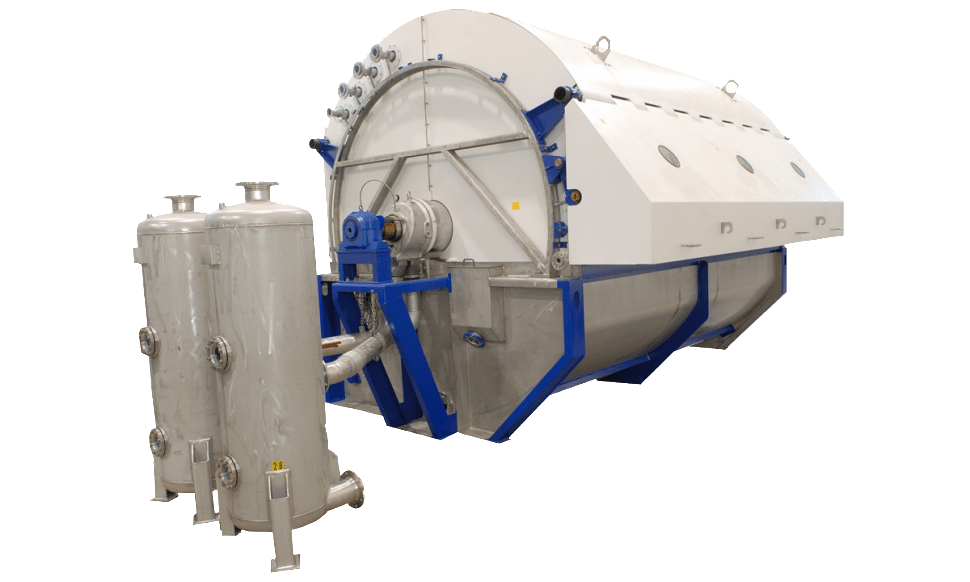# Basic Filtration Equation

Let’s learn the basic filtration equation which may be useful in sizing and evaluating many types of filtration unit. Disclaimer, this is also the first time in my working life learning about filtration equation, so if you find any mistakes, please feel free to comment below.

There are two terms in basic filtration equation. The first is, if the filtration occurs at constant pressure. And the second is, if the filtration occurs at constant flow rate. If the filtration occurs at constant pressure, then the flow rate will progressively diminished because the filter bed is steadily growing in thickness. Whereas, if the filtration occurs at constant flow rate, then the pressure must be gradually increased.

Because the particles forming the cake are small and the flow through the bed is slow, streamline conditions are almost always obtained, therefore the flow rate of filtrate can be represented by the following equation: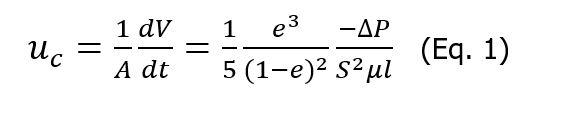Where:

V = volume of filtrate which has passed in time t

A = total cross-sectional area of the filter cake

uc = superficial velocity of the filtrate

l = cake thickness

S = specific surface of the particles

e = voidage

μ = viscosity of the filtrate

∆P = applied pressure difference

Equation above is derived by assuming that the cake is uniform and the voidage is constant throughout.

Filter cakes may be divided into incompressible cakes and compressible cakes.

Read other posts related to filtration process: A Simple Calculation on How to Determine Time Required to Obtain Certain Volume of Filtrate.

For an incompressible cake, the resistance to flow for a given volume of cake is not significantly affected by either pressure difference across the cake or by the rate of material deposition. On the other hand, with a compressible cake, increase in pressure difference or flow rate causes the formation of a denser cake with a higher resistance.

For incompressible cakes, e (voidage) in Eq. 1 may be taken as constant, and the quantity e3/[5 (1-e)2 S2] is then a property of the particles forming the cake and should be constant for a given material.

Therefore: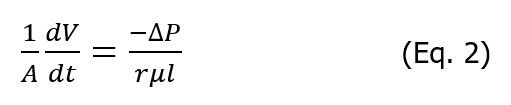Where: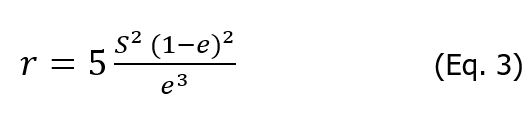Eq. 2 is a basic filtration equation, and r is specific resistance which is a function of S (specific surface of particles) and e (voidage). For incompressible cakes, r is taken as constant, although it depends on the rate of deposition, the nature of the particles, and on the forces between the particles. Dimension or r is m-2 in the SI system.

## Relation Between Thickness of Cake and Volume of Filtrate

In Eq. 2, the variables of l (cake thickness) and V (volume of filtrate) are connected. The relation between them may be obtained by making material balance between solids in both slurry and the cake. This is the material balance:

Mass of solid in filter cake = (1-e) Alρs, where ρs is the density of the solids.

Mass of liquid retained in the filter cake = eAlρ, where ρ is the density of the filtrate.

If J is the mass fraction of solids in the original suspension then: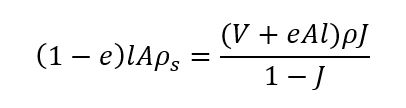orTherefore: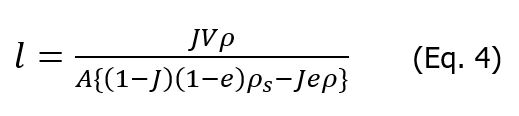and:If v is the volume of cake deposited by unit volume of filtrate, then: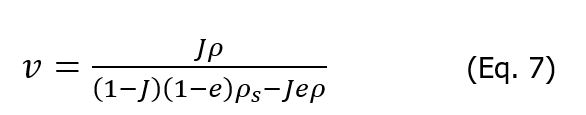Substituting for l in Eq. 2:Where:

V = volume of filtrate which has passed in time t

A = total cross-sectional area of the filter cake

l = cake thickness

μ = viscosity of the filtrate

∆P = applied pressure difference

v = volume of cake deposited by unit volume of filtrate

r = specific resistance

Eq. 8 is basic filtration equation which relates –∆P, V, and t.

As mentioned above, there are two important operation type of filtration, where the pressure difference is maintained constant, and where the filtration rate is maintained constant.

For a filtration at constant flow rate:Therefore,orFrom Eq. 10 we can see that -∆P is directly proportional to V.

For a filtration at constant pressure difference, Eq. 8 may be integrated: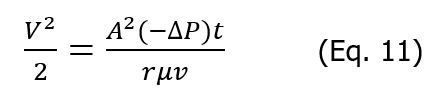orBased on Eq. 12, for a constant pressure filtration, there is a linear relation between V2 and t or between t/V and V.

Filtration at constant pressure is more frequently applied in practice, although the pressure difference is normally gradually built up to its ultimate value.

If this takes a time t1 during which a volume V1 of filtrate passes, then integration of Eq. 8 gives:or: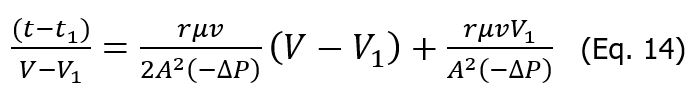t-t1 represents the time of the constant pressure filtration and (V-V1) correspond volume of filtrate obtained.

## Flow of Filtrate Through the Cloth and Cake Combined

If the filter cloth and the initial layers of cake are together equivalent to a thickness L of cake, as deposited at a later stage in the later process, and if -∆P is the pressure drop across the cake and cloth combined, then:Where:

L = thickness of filter cloth and the cake at a later stage

Eq. 16 may be integrated with the following limits.

For constant filtration flow rate:

t = 0, V = 0

t = t1, V = V1

For constant pressure filtration

t = t1, V = V1

t = t, V = V

For the period of constant rate filtration: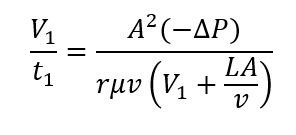or: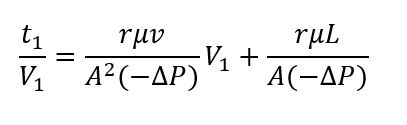or: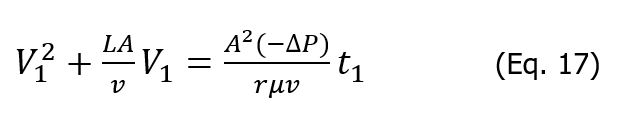For a subsequent constant pressure filtration: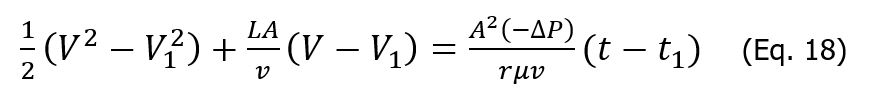or: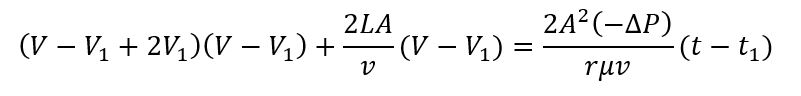or:Thus, there is linear relation between (t-t1)/(V-V1) and VV1 and the slope is proportional to the specific resistance.

The intercept on the (t-t1)/(V-V1) axis should enable L, the equivalent thickness of the cloth, to be calculated.

Reference:

Coulson and Richardson’s Chemical Engineering Volume 2A, Sixth Edition, 2019.